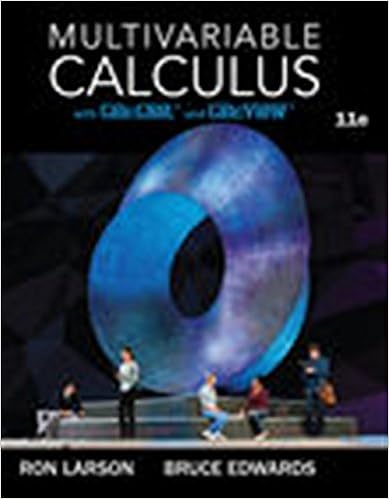# 2007Test3Solutions - MATH 2007B Test 3 Solutions[Marks[8[10...

• Test Prep
• 2
• 100% (2) 2 out of 2 people found this document helpful

This preview shows page 1 - 2 out of 2 pages.

##### We have textbook solutions for you!
The document you are viewing contains questions related to this textbook.The document you are viewing contains questions related to this textbook.
Chapter 12 / Exercise 70
Multivariable Calculus
LarsonExpert Verified
MATH 2007BTest 3 SolutionsNovember 11, 2016[Marks]1. Evaluate the improper integral or show that it diverges.(a)integraldisplay0e-xdx(b)integraldisplay11x1/3dx(c)integraldisplay101x1/3dx(d)integraldisplay1-11xdxSolution:
2. Consider the parametric curvex=32t2,y=t3-3t2.(a) Finddydx.(b) Determine the point(s) (x, y) where the tangent is horizontal.(c) Find the equation of the tangent line att= 1.(d) Determine the interval(s) ofton whichyis increasing, and the interval(s) onwhichyis decreasing.(e) Findd2ydx2.
2
##### We have textbook solutions for you!
The document you are viewing contains questions related to this textbook.The document you are viewing contains questions related to this textbook.
Chapter 12 / Exercise 70
Multivariable Calculus
LarsonExpert Verified
2
3. Find the length of the parametric curvex= 1-t3,y= 2 +32t2, 0t3.
•••